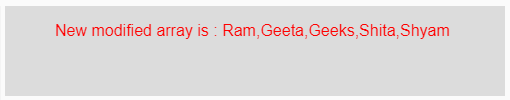Open in App
Not now

# p5.js | splice() function

• Last Updated : 10 Feb, 2021

The splice() function in p5.js is used to insert values or array elements in the given array.
Syntax:

`splice(Array, Values, Position)`

Parameters: This function accepts three parameters as mentioned above and described below:

• Array: This is a given array which is to be modified.
• Values: This is the values or an another array whose elements are to be inserted into the given array which are to modified.
• Position: This is the number of elements of the given array after which new values or elements of another array are to be inserted.

Return Value: It returns a new modified array.
Below programs illustrate the splice() function in p5.js:
Example 1: This example uses splice() function to insert values or array elements in the given array.

## javascript

 `function` `setup() {` `    ``// Creating Canvas size``    ``createCanvas(500, 90);``}` `function` `draw() {``    ` `    ``// Set the background color``    ``background(220);``    ` `    ``// Initializing the array``    ``let Array = [9, 6, 0, 22, 4, 1, 15];``  ` `    ``// Initializing the value which are``    ``// to be inserted``    ``let Value = 5;``  ` `    ``// Initializing the position after``    ``// which insertion is done``    ``// counting is done from starting``    ``let Position = 5;``  ` `    ``// Calling to splice() function.``    ``let A = splice(Array, Value, Position);``    ` `    ``// Set the size of text``    ``textSize(16);``    ` `    ``// Set the text color``    ``fill(color(``'red'``));``  ` `    ``// Getting new modified array``    ``text(``"New modified array is : "` `+ A, 50, 30);          ``}`

Output:Example 2: This example uses splice() function to insert values or array elements in the given array.

## javascript

 `function` `setup() {` `    ``// Creating Canvas size``    ``createCanvas(500, 90);``}` `function` `draw() {``    ` `    ``// Set the background color``    ``background(220);``    ` `    ``// Initializing the array``    ``let Array = [``'Ram'``, ``'Geeta'``, ``'Shita'``, ``'Shyam'``];``  ` `    ``// Initializing the value which are``    ``// to be inserted``    ``let Value = ``'Geeks'``;``  ` `    ``// Initializing the position after``    ``// which insertion is done``    ``// counting is done from starting``    ``let Position = 2;``  ` `    ``// Calling to splice() function.``    ``let A = splice(Array, Value, Position);``    ` `    ``// Set the size of text``    ``textSize(16);``    ` `    ``// Set the text color``    ``fill(color(``'red'``));``  ` `    ``// Getting new modified array``    ``text(``"New modified array is : "` `+ A, 50, 30);          ``}`

Output:Example 3: This example uses splice() function to insert array elements into another array.

## javascript

 `function` `setup() {` `    ``// Creating Canvas size``    ``createCanvas(600, 90);``}` `function` `draw() {``    ` `    ``// Set the background color``    ``background(220);``    ` `    ``// Initializing the array``    ``let Array = [``'Ram'``, ``'Geeta'``, ``'Shita'``, ``'Shyam'``];``  ` `    ``// Initializing a new array whose elements are``    ``// to be inserted``    ``let Value = [``'Geeks'``, ``'GeeksforGeek'``];``  ` `    ``// Initializing the position after``    ``// which insertion is done``    ``// counting is done from starting``    ``let Position = 2;``  ` `    ``// Calling to splice() function.``    ``let A = splice(Array, Value, Position);``    ` `    ``// Set the size of text``    ``textSize(16);``    ` `    ``// Set the text color``    ``fill(color(``'red'``));``  ` `    ``// Getting new modified array``    ``text(``"New modified array is : "` `+ A, 50, 30);``             ` `}`

Output:Reference: https://p5js.org/reference/#/p5/splice

My Personal Notes arrow_drop_up